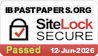# Topic 6: Circular motion and gravitation

## 6.1 – Circular motion

• Period, frequency, angular displacement and angular velocity

Uniform circular motion refers to circular motion at constant speed.

In a uniform circular motion, speed is constant while (angular) velocity and (angular) acceleration are constantly changing.

• While the magnitude of its velocity remains constant, the direction of its velocity is constantly changing.
• The acceleration causing this change in velocity is always directed towards the center of the circular path.

The period is the time taken for the object to complete one full circle and is usually calculated in seconds. The frequency can be calculated by 1/period and is usually measured in Hz.

• Centripetal force

Centripetal force is the corresponding force (resultant force) which causes the centripetal acceleration.

Properties:

• Direction: Pointing towards the center of the circle / perpendicular to the instantaneous velocity
• Magnitude:
• Work done by centripetal force = 0
• Centripetal force is not a type of force; rather, it is just the name we give to the net force causing a circular motion. For example:
• Centripetal acceleration

The acceleration which gives rise to a circular motion is called the centripetal acceleration. Its magnitude is given by

It is directed towards the center of the circular motion and is perpendicular to the instantaneous velocity of the object.

## 6.2 – Newton’s law of gravitation

• Newton’s law of gravitation

The gravitational force between two objects can be calculated using Newton’s universal law of gravitation

• Gravitational field strength

The gravitational field strength at a point is the force per unit mass experienced by a test mass at that point.

The gravitational field strength (g) due to an object is given by

Gravitational field strength at the surface of a planet

• The gravitational field strength at the surface of a planet can be calculated by using the equation for gravitational field strength and substituting M and r by the mass and the radius of the planet respectively.
• If we calculate the gravitational field strength at the surface of the Each using the mass and the radius of the Earth, we would obtain the value 9.81m/s^2, which is equal to the acceleration due to gravity on the surface of the Earth.
• Different planets have different radii and masses. Consequently, different planets have different gravitational field strengths.

Topic 6: Circular motion and gravitation